UPSC  >  Interests: Solved Examples- 1

# Interests: Solved Examples- 1 - CSAT Preparation - UPSC

 Table of contentsInterest, Principal, Amount, Rate & TimeCompound InterestDifference between CI & SISome Solved Examples on InterestShares & StocksSome Solved Examples of Stocks & Shares## Interest, Principal, Amount, Rate & Time

### Interest

If person A borrows some money from another person B for a certain period, then after that specified period, the borrower has to return the money borrowed as well as some additional money. This additional money that borrower has to pay is called interest.

### Principal

The actually borrowed money by A is called principal (SUM).

### Rate

The interest that the borrower has to pay for every 100 rupees borrowed for every year is known as rate percent per annum. It is denoted as R% per annum =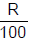### Time

The time for which the borrowed money has been used is called the time. It is denoted as T years.

### The Formula of Simple Interest

The interest is directly proportional to the principal, the rate and time for which the borrowed sum is used. If the interest on a certain sum borrowed for a certain period is reckoned uniformly, then it is called Simple Interest and denoted as S.I.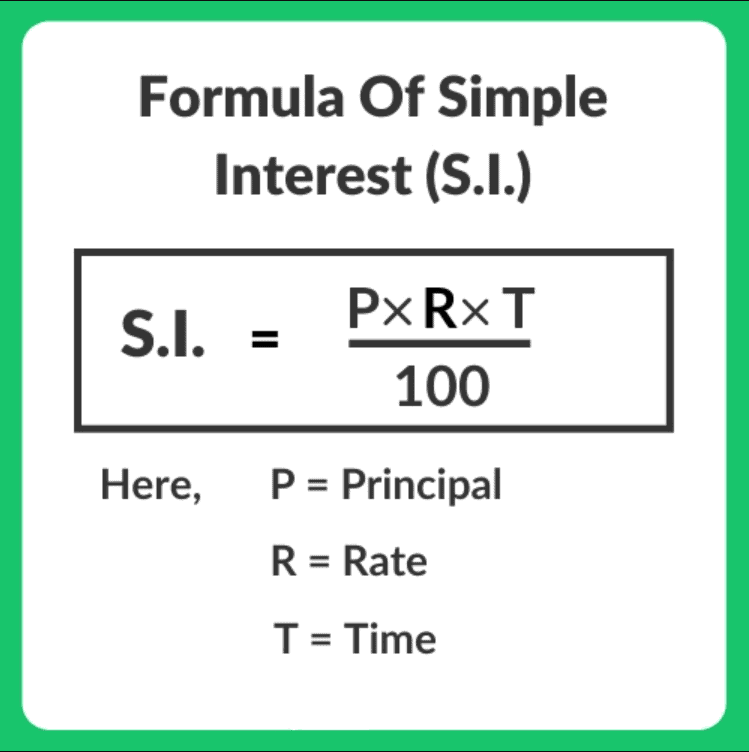Where P = Principal or the sum borrowed

R = Rate percent per annum

T = Number of years for which the borrowed money has been used.

### Amount

The principal and the interest together is called amount.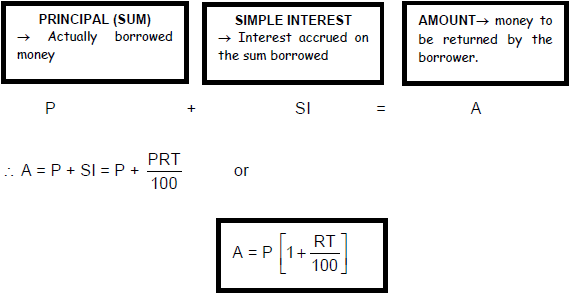Question for Interests: Solved Examples- 1
Try yourself:A certain sum of money invested at some rate of interest triples itself in 4 years. In how many years the principal will become 9 times of itself at the same rate?

Example on Repayment Of Debt In Equal Installments

Dr. M. I. Rajpoot wants to buy an air-conditioner for his family. He went to a shop and selects an AC whose price is Rs. 12,700. The shopkeeper gave him two offers either you pay the full amount of Rs. 12,700 or pay only Rs. 4,000 and installment of Rs. 3000 per month for next 3 months just paying only Rs. 300 as interest. What is the rate of interest the shopkeeper charged Rajpoot?

Sol. In these types of problems the interest charged is always calculated on the basis of the one-month principal, not on the amount of the loan taken. Here first of all we have to calculate the one-month principal for every installment paid.

Principal for 1st month = loan amount = Rs 8,700
Principal for 2nd month = loan amount – 1st installment = Rs (8,700 – 3,000) = Rs. 5,700

Principal for 3rd month = Rs. (5,700 – 3,000) = Rs. 2,700

Total one month principal = Rs.(8700 + 5700 + 2700) = Rs. 17,100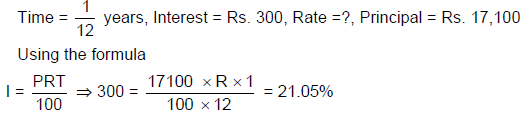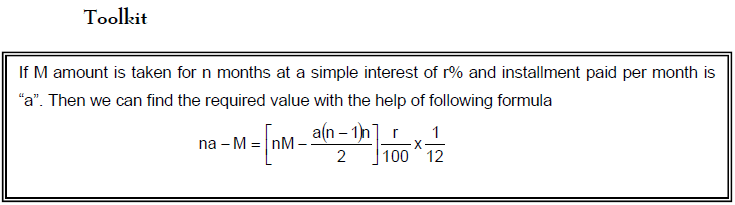Compound Interest

As discussed in the topic on ‘Simple Interest’, the principal (P) remains constant throughout the period for which the money (principal) is borrowed.

• But, in the case of compound interest, the total interest received in the present year will be added to the original principal and for the following year, the principal will be Amount Received (Principal + interest).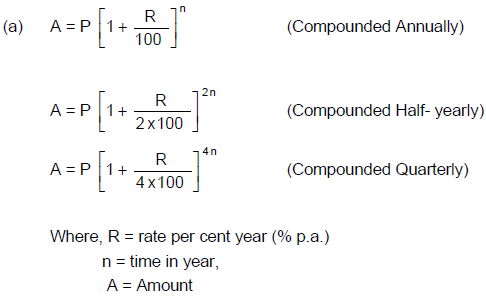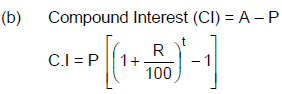Question for Interests: Solved Examples- 1
Try yourself:A certain sum of money doubles in 3 years, then in how many years it will become 8 times at compound interest?

## Difference between CI & SI

For the first year the simple interest and compound interest, both are same, but with the next following years the C.I will always be more than the S.I.

Let P is the principal invested at r% rate per annum at S.I and C.I respectively. What will be the difference between SI and CI for different years?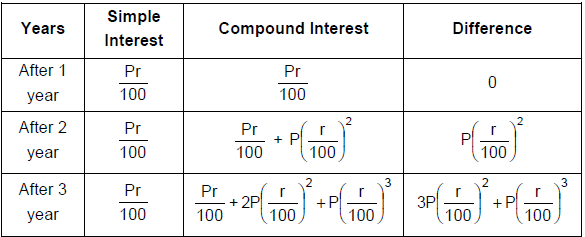• Hence for every changing year, the principal goes on changing, and accordingly, the amount of interest accrued on varying principal will be different every year.
• The money lent under this condition is charged with Compound Interest. While solving the problems on Compound Interest, it is assumed that Interest is compounded yearly, unless otherwise specified.

### Equal Annual Installment

Let the value of each equal annual installment = Rs. A
Rate of interest = R % p.a. at CI
Number of installments per year = n
Number of years = T
∴ Total number of installments = n × T
Borrowed Amount = B
Then,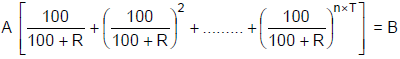## Some Solved Examples on Interest

Ex.1 If a principle becomes 3 times in 10 years according to simple interest, what is the rate of interest?

Sol. Principle becomes 3 times means, the interest is 2P.

∴ 2P =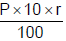⇒ r = 20%

Ex.2 A man took Rs. 5000 at 10% simple interest and gave it to another person at 10% compound interest, which is being compounded annually. After 3 years, how much extra money he will get?

Sol. He has to pay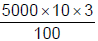= 6500
He will get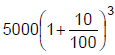= 6655
So, the ans is 6655 – 6500 = Rs 155.

Ex.3 Certain money becomes double in 4 years according to simple interest. In how many years, will it become 3 times?

Sol. If P is the principle, it becomes 2P in 4 years, that means the interest earned is 2P – P = P. If it has to become 3 times means the interest has to become 2P, so, it takes double the time. i.e. 2 × 4 = 8 years.

Ex.4 Certain money doubles itself according to compound interest in 3 years. How much time does it take to become 4 times?

Sol. Since the money gets doubled in 3 years according to the Compound Interest, it again gets doubled in the next 3 years. So it gets 4 times in 6 years.
∴n = 6 years.

Ex.5 Mr. A deposited certain amount in a bank exactly 5 years back @ of 8.8% simple interest. Mr. B deposited the same amount of money exactly 2 years back, which is for compound interest, compounded annually. Now both they got same amount of money at what rate of interest, Mr. B deposited his money.

Sol. Mr. A will get P +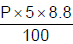= 1.44P
Mr. B will get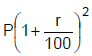Since both got the same money.= 1.44P
⇒ r = 20%

Ex.6 On a sum of Rs. 1000, the C.I. for 2 years is twice the S.I. for 2 years when the rate is 11%. Find the rate at which the interest is compounded annually?

Sol. S.I. at 11% for 2 years on a sum of Rs. 1000 = 220
Since C.I. is twice the S.I.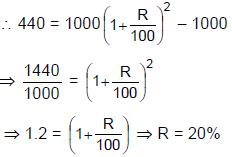Ex.7 Two equal sums are lent at the same time at 6% and 5% simple interest respectively. The former is received 2 years earlier than the later, and the amount in each case is Rs. 2400. Find the sum?

Sol. Let the sum be Rs. x & the latest period be n years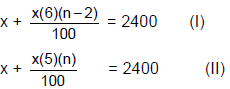Solving these two equations simultaneously,
n = 12, x = 1500 The periods are 10 years & 12 years, Sum is Rs. 1500.

Ex.8 Ram invests a certain amount of money and earns a Compound Interest of Rs. 420 in the second year and a C.I. of Rs. 462 in the third year. Calculate at what rate of interest did Ram invest?

Sol. C.I. on third-year – C.I. on second-year = 462 – 420 = 42
Thus Rs. 42 is the interest on Rs. 420.  i.e. 10% of 420

Hence Rate = 10%.

Ex.9 Robin lend out Rs. 9 on the condition that the loan is payable in 10 months by 10 equal installments of Re. 1 each. Find the rate of interest per annum.
Sol. Let the rate of interest per month be r
Total amount repaid = Rs.10 interest = Re. 1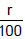(9 + 8 + 7 + 6 + 5 + 4 + 3 + 2 + 1) = 1
r =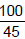Rate of interest per annum = (100/45) 12 = 26 2/3%.

## Shares & Stocks

### Shares

The capital is divided into equal parts called shares.

• Shares may be of any value from Rs. 10 (or less) up to Rs. 100 (or more). Each person who purchases shares is called a shareholder and becomes a part-owner of the company.
• He gets a share certificate indicating the number of shares he holds in the company.
• Sometimes a company issues a certificate called the stock certificate instead of a share certificate indicating that a person holds a stock of say Rs. 5000 i.e. shares worth Rs. 5000.
• The value of a share mentioned in the share certificate is called its nominal value, face value or par value (nominal value does not indicate its cash value).
• The nominal value is a fixed quantity, but the cash value may vary from hour to hour. The price of a share at any particular time is called its market value.
• Shares can be preferred shares or ordinary shares.

##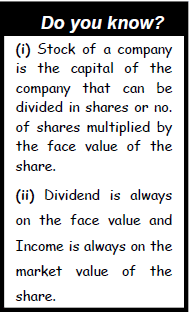Stock

The term stock is also applied to the various amounts of money which have been borrowed by the Government or by the corporations of cities.

To pay the interest on different sums of money borrowed from time to time, the Government sets aside certain funds.

Kinds of Stock

(1) Stock at Par: Stock is said to be at par if the market value of the stock is equal to its face value. (Market Value = Face Value)

(2) Stock at a premium (or above par): Stock is said to be at a premium if the market value of the stock is greater than its face value. (Market value = Face value + Premium)

(3) Stock at a discount (or below par): Stock is said to be at a discount if the market value of the stock is less than its face value. (Market value = Face value – discount)

For example, If Rs. 100 stock is bought for Rs. 106, the stock is at a premium of 6% or 6 above par; but if it is bought for Rs. 96, the stock is at a discount of 4% or 4 below par; and if Rs. 100 stock is bought for Rs. 100, it is said to be at par.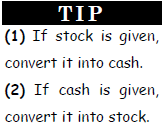The distinction between Stock & Shares

The total investment of a company is called stock.

• This stock is divided into a number of shares of equal face value.
• The total face value of a number of shares is also stock. For example, 100 shares of Rs. 10 each is Rs. 1000 stock and 10 shares of Rs. 100 each is also Rs. 1000 stock.
• Clearly, a share is also a stock, but the stock may not be a share, for it may consist of more than one share.

### Dividend

When a company makes a profit, part of that profit is divided amongst the shareholders and it is called the dividend. The dividend is always calculated on the face value of a share and is generally expressed as a percentage.

### Brokerage

The stock is generally bought or sold through a broker who charges a small commission called the brokerage. A buyer has to pay the market value together with the brokerage and a seller gets market value reduced by the brokerage i.e.

Amount paid by the buyer = Market value + Brokerage.

Amount received by the seller = Market value – Brokerage.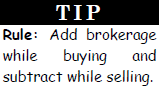### Debentures

A debenture is an acknowledgement of a debt of a company. Debentures are equal parts of a loan raised by a company. A debenture holder is a creditor of the company and is entitled to a fixed return every year irrespective of profits. If a company is wound up, the amount due to debenture holders must be paid before anything is paid to the share holders.

## Some Solved Examples of Stocks & Shares

Ex.1. How much stock can be purchased with Rs. 52,625 at 5% above par (Brokerage ¼ %).

Sol. To purchase Rs. 100 stock we need Rs.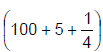= Rs.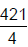If investment is Rs.; stock purchased = Rs. 100.

If investment is Rs. 52,625; stock purchased = 100 ×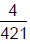× 52,625 = Rs. 50,000.

Ex.2. A man buys Rs. 6000 stock at 5% discount and sells at 2% above par. Find his gain or loss. Brokerage @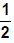%.
Sol.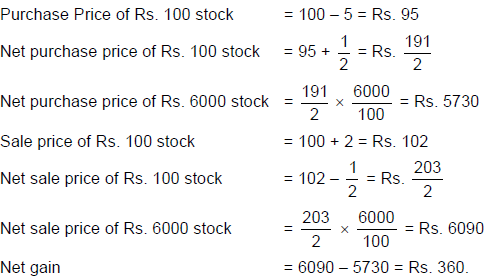Alternate Method: From above –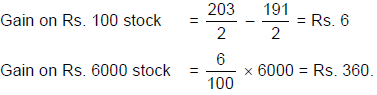Income Problem
A statement such as ‘5% stock @ 95’ means.

1. Face value of stock is Rs. 100.

2. Market value of Rs. 100 stock is Rs. 95.

3. Income (Annual) from this stock is Rs. 5.
Hence we get Rs. 5 as income or dividend (Annual) by investing Rs. 95 and obtain a stock worth Rs. 100.

Note: Percentage Return on the shares purchased less than the market value will always be more than the actual return.

Ex.3. X invested an amount of Rs. 23,920 in 8% stock at 92. Find his net income if he pays 4% income tax.
Sol. Cost of Rs. 100 stock = Rs. 92
Income on Rs. 92 = Rs. 8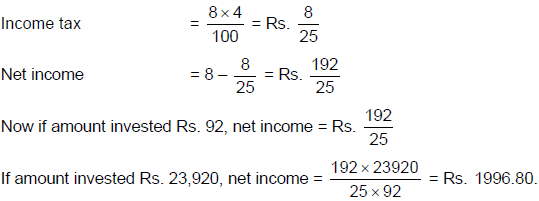The document Interests: Solved Examples- 1 | CSAT Preparation - UPSC is a part of the UPSC Course CSAT Preparation.
All you need of UPSC at this link: UPSC

## CSAT Preparation

197 videos|151 docs|200 tests

## FAQs on Interests: Solved Examples- 1 - CSAT Preparation - UPSC

 1. What is compound interest?Compound interest refers to the interest that is calculated not only on the initial amount (principal) but also on the accumulated interest from previous periods. In other words, it is the interest earned on both the principal and the previously earned interest.
 2. How is compound interest different from simple interest?The key difference between compound interest (CI) and simple interest (SI) lies in the calculation of interest. Compound interest takes into account the interest earned in previous periods and adds it to the principal, whereas simple interest is calculated solely on the principal amount. As a result, compound interest tends to yield higher returns compared to simple interest over time.
 3. Can you provide an example to understand compound interest better?Certainly! Let's consider an example. Suppose you invest \$1,000 in a fixed deposit account with an annual interest rate of 5%. If the interest is compounded annually, the interest earned in the first year would be \$50 (\$1,000 * 0.05). In the second year, the interest would be calculated on the new principal amount, which is \$1,050 (\$1,000 + \$50). Therefore, the interest earned in the second year would be \$52.50 (\$1,050 * 0.05). This process continues for each subsequent year, resulting in the compounding of interest.
 4. What are shares and stocks?Shares and stocks are terms used to represent ownership in a company. A share refers to a single unit of ownership, while stocks represent the collective ownership of all shares in a company. When you own shares or stocks of a company, you become a shareholder and have certain rights, such as voting rights and receiving dividends.
 5. Can you provide an example related to shares and stocks?Certainly! Let's say you purchase 100 shares of XYZ Company at \$10 per share. In this case, you own stocks worth \$1,000 (100 shares * \$10 per share). If the value of the stocks increases to \$15 per share, your stocks would be worth \$1,500 (100 shares * \$15 per share). By selling the stocks at this higher price, you would make a profit of \$500 (\$1,500 - \$1,000). This example demonstrates the potential for gaining returns through investing in shares and stocks.

## CSAT Preparation

197 videos|151 docs|200 testsExplore Courses for UPSC exam### How to Prepare for UPSC

Read our guide to prepare for UPSC which is created by Toppers & the best Teachers
Signup to see your scores go up within 7 days! Learn & Practice with 1000+ FREE Notes, Videos & Tests.
10M+ students study on EduRev
Track your progress, build streaks, highlight & save important lessons and more!
Related Searches

,

,

,

,

,

,

,

,

,

,

,

,

,

,

,

,

,

,

,

,

,

;